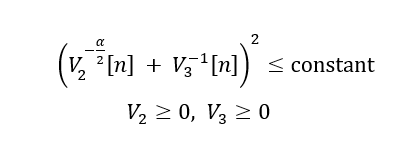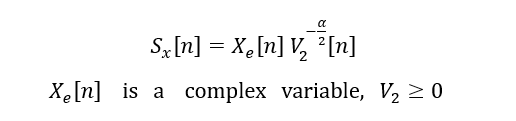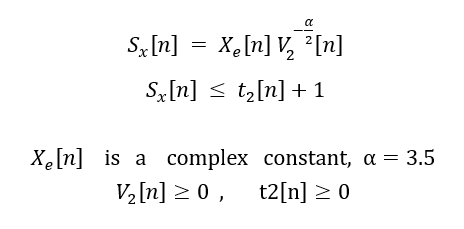# Square() and pow_pos()

Hello, everyone!
I express the constraint as follows, I want to know whether it is right?

``````square( pow_pos( V2(n),-a/2)  + pow_pos(V3(n),-1)  ) <=constant
``````Use `pow_p`, not `pow_pos`. For the 2nd term, you also could use `inv_pos`

help pow_pos

pow_pos Power of positive part.
pow_pos(X,P) = POS(X).^P = MAX(X,0).^P.
Both P and X must be real, and P must be greater than or equal to 1.

``````Disciplined convex programming information:
pow_pos(X,P) is convex and nondecreasing in X; so when used in CVX
expressions, X must be convex. P must be constant, real, and
greater than or equal to 1.
``````

help pow_p
pow_p Positive branch of the power function.
pow_p(X,P) computes a convex or concave branch of the power function:
P < 0: pow_p(X,P) = X.^P if X > 0, +Inf otherwise
0 <= P < 1: pow_p(X,P) = X.^P if X >= 0, -Inf otherwise
1 <= P : pow_p(X,P) = X.^P if X >= 0, +Inf otherwise
Both P and X must be real.

``````Disciplined convex programming information:
The geometry of pow_p(X,P) depends on the precise value of P,
which must be a real constant:
P < 0: convex  and nonincreasing; X must be concave.
0 <= P < 1: concave and nondecreasing; X must be concave.
1 <= P    : convex  and nonmonotonic;  X must be affine.
In all cases, X must be real.
``````

But have another problem about ‘‘Cannot perform the operation: {complex affine} . {convex}*’’. The constraint and my code are as follows:

Sx(n) = Xe(n) * pow_p( V2(n) , - alpha ) ;Sorry, `Xe[n] is a complex constant`

That is non-convex. it would be non-convex (neither convex nor concave) even if `X` were real rather than complex. It would be non-convex, even if `V` were not raised to any power.

I don’t know how `S` is used in your model, so I can’t conclude that the model is non-convex. Perhaps there is a way of reformulating the code so as to never form `S`, but quite possibly, such a reformulation is not possible.

Thank you! how to use `S` in my model is as follows :I replace `Xe[n]` as `2` , it not report the error.

If `Xe(n)` is a positive constant, that is a convex inequality. If it is not, that does not look like a convex inequality. Please carefully read the link in my previous post.